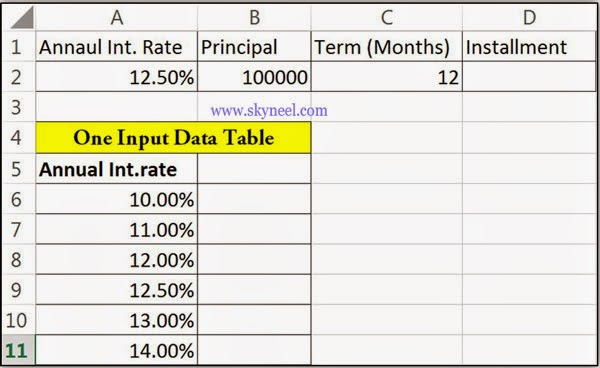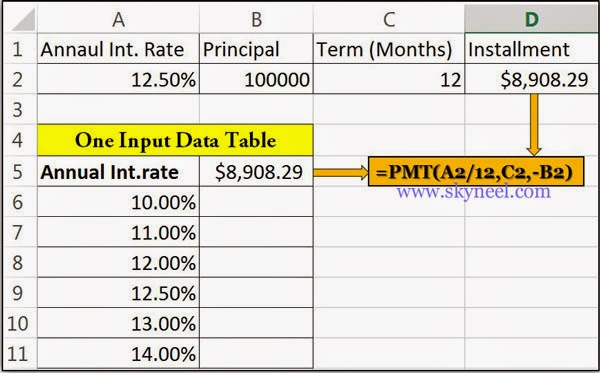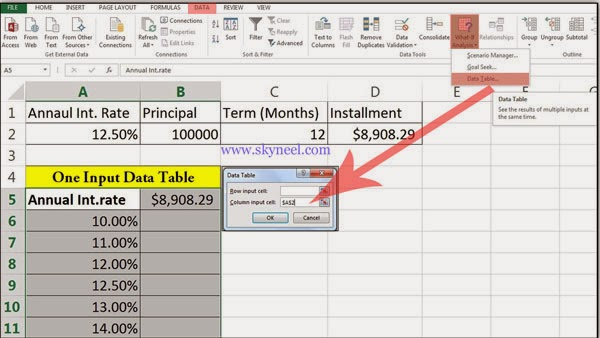# What-If Analysis and Excel’s One Input Data Table

“One-Input Data Table” facility which is part of the What-If Analysis of Excel. Method of modify the cell values and see effect of modifications .

Today we discuss an important “One-Input Data Table” facility which is part of the What-If Analysis of MS Excel. One-Variable Data table allows you to modify only single variable value in your sheet and view how changing the values for that variable affect the values of calculated by one or more formulas in active sheet.

### What-IF Analysis:

It is a method of modify the cell values and see how these modifications are taking affect the result of formulas on the worksheet. For example, varying the interest rate and term that is used in an amortization table to determine the amount of the payments.

#### Data Table:

It is a part of the What-If-Analysis facility which is used to change one or more than one variables values and watch the result.

#### Formula:

A series of numbers, reference of any cell, use of functions or any operators which is used in a cell that provide the final resulted value. In MS Excel, a formula always starts with an equal sign (=) operator. You can create one-input data tables from what if analysis facility in MS Excel, to change the value of only one variable to view the effect on the resulted area.

For Example:
If you take loan from any bank on different interest rate and for different periods in months and you want to know how changing interest rate percentage variable affect the installment amount in active sheet, then you can use one input data table facility in MS Excel.

### How to create 1-Input Data Table

Step 1: If you want to create one input data table then first, you have to prepare following given sheet:Step 2: Type following formula in Cell D2 and B5:
=PMT(A2/12,C2,-B2)   then press enter key to view the result. Excel shows \$ 8908.29 as a result.Step 3: Select the range from A5:B11 -> Click Data Tab -> What-If Analysis -> Data Table -> Type \$A\$2 in Column Input Cell  box in Data Table dialog box. -> Click OK button.Step 4: Now, you will see the final result in one input data table: